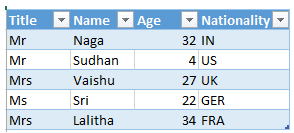## Vba tables in vba in Excel

The Excel tables are accessed in VBA using the ListObjects Object.

The "Listobjects" returns a "Listobject" which represents an Excel Table.

However, "Listobjects" is used to access an already existing table.

Create Table

The following example shows, how to create a new table in excel.

1. Sub CreateNewTable()
2. Sheets("Q49").ListObjects.Add(xlSrcRange, Range("K1:N10"), , xlYes).Name = "myTable1"
3. End Sub

The above example creates a plain table as shown in the right.

Convert to Range

The following code shows how to convert the table to Normal range.

1. Sub tabletorange()
2. On Error Resume Next
3. Sheets("Q49").ListObjects("myTable1").Unlist
4. End Sub

Loop through Table

The following code shows loops through each Table column/row

1. Sub tableloop()
2. Dim tbl As ListObject
3. Dim x As Long
4. Set tbl = ActiveSheet.ListObjects("myTable1")
5. On Error Resume Next
6. 'Loop Through Each Column in Table
7. For x = 1 To tbl.ListColumns.Count
8. tbl.ListColumns(x).Range.ColumnWidth = 8
9. Next x
10. 'Loop Through Every Row in Table
11. For x = 1 To tbl.Range.Rows.Count
12. tbl.Range.Rows(x).RowHeight = 20
13. Next x
14. 'Loop Through Each DataBody Row in Table
15. For x = 1 To tbl.ListRows.Count
16. tbl.ListRows(x).Range.RowHeight = 15
17. Next x
18. End Sub

Clear Filter in Table

The following code shows how to clear filter in Table headers

1. Sub sbClrFtr()
2. If Sheets("Q49").FilterMode = True Then
3. Sheets("Q49").ListObjects("myTable1").Range.AutoFilter
4. End If
5. End Sub

A screeenshot of the sample table is shown below